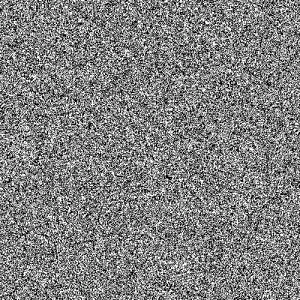October 19, 2021

# Creating visually random images

How complicated does a mathematical function, pseudorandom(x), have to be to create something that seems random, and how do you check whether it seems random? Looking at a long list of numbers is not a good way, because our ability to look at long lists of numbers is very limited. Our ability to look at images is much better.

So, to inspect whether a list of numbers is seemingly random, a fun way is to create an image using the numbers for each pixel, and simply look at it.

Given a number x in the series {0, 1, 2, ..., huge n}, let's create an image that plots a random function that's implemented using the sin() function and a 3rd degree polynomial function:

```color_range = 2**8 a = -3.0 b = -5.0 c = 13.0   def pseudo_randombit(x): color255 = math.sin(a*x**3 + b*x**2 + c*x) % color_range # make black/white bit = color255 / 127 return bit```

I'm no signal processing expert, but his approach intuitively seems similar to distortion. Create a big number and make it fit inside a small room, throwing sin() into the mix for to make the number flip between positive and negative number. Note: I should refine the function given this realization, and simply check the sign...

The full example that uses GDAL (sinpol3_raster.py). Nevermind the geographical projection stuff, I copy-pasted some code, sorry:

```import osr import numpy import gdal import math import sys   color_range = 2**8 a = -3.0 b = -5.0 c = 13.0   def pseudo_randombit(x):   color255 = math.sin(a*x**3 + b*x**2 + c*x) % color_range # make black/white bit = color255 / 127 return bit   def main(argv):   width = int(argv) height = int(argv)   format = "GTiff" driver = gdal.GetDriverByName( format )   dst_ds = driver.Create( "sinpol3.tif", width, height, 1, gdal.GDT_Byte )   dst_ds.SetGeoTransform( [ 444720, 30, 0, 3751320, 0, -30 ] )   srs = osr.SpatialReference() srs.ImportFromEPSG(25832) dst_ds.SetProjection( srs.ExportToWkt() )   raster = numpy.zeros( (height, width), dtype=numpy.uint32 )   for x in range(0, height*width-1):   bit = pseudo_randombit(x) # make black/white color = bit * 255 i = (x / width) j = x % width raster[i][j] = color   dst_ds.GetRasterBand(1).WriteArray( raster )   if __name__ == '__main__': main(sys.argv)```

Calling sinpol3_raster.py:

```\$ python sinpol3_raster.py 500 500 \$ ls *.tif sinpol3.tif```

sinpol3.tif:Close-up of sinpol3.tif:An idea that takes this idea further is cryptographic QR-codes... Is the pseudo-random function given above directly reversible? I have to think about this. But now I have other matters to attend to, like a phd in high-availability geospatial services.#### kostas

View all posts by kostas →

This site uses Akismet to reduce spam. Learn how your comment data is processed.